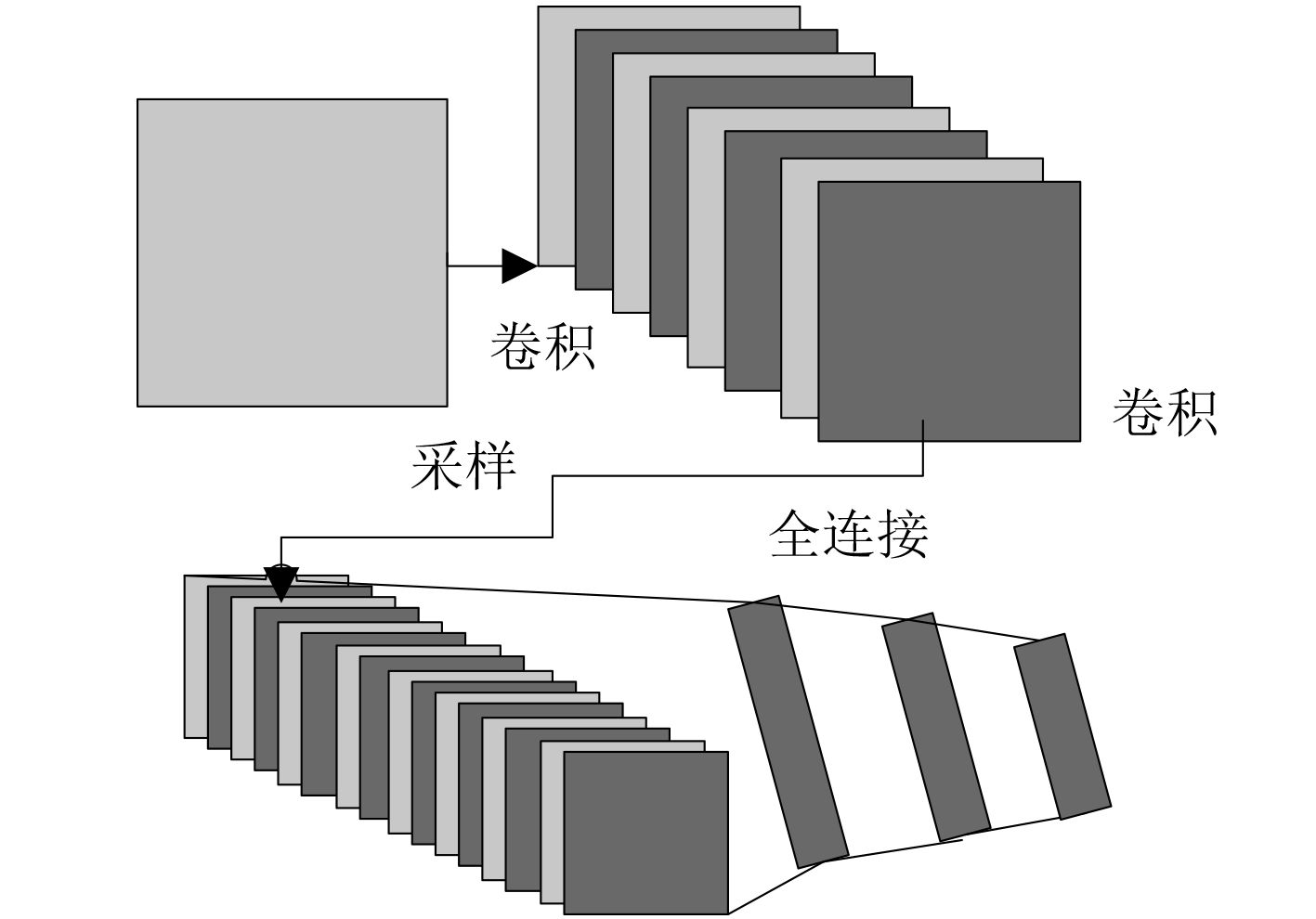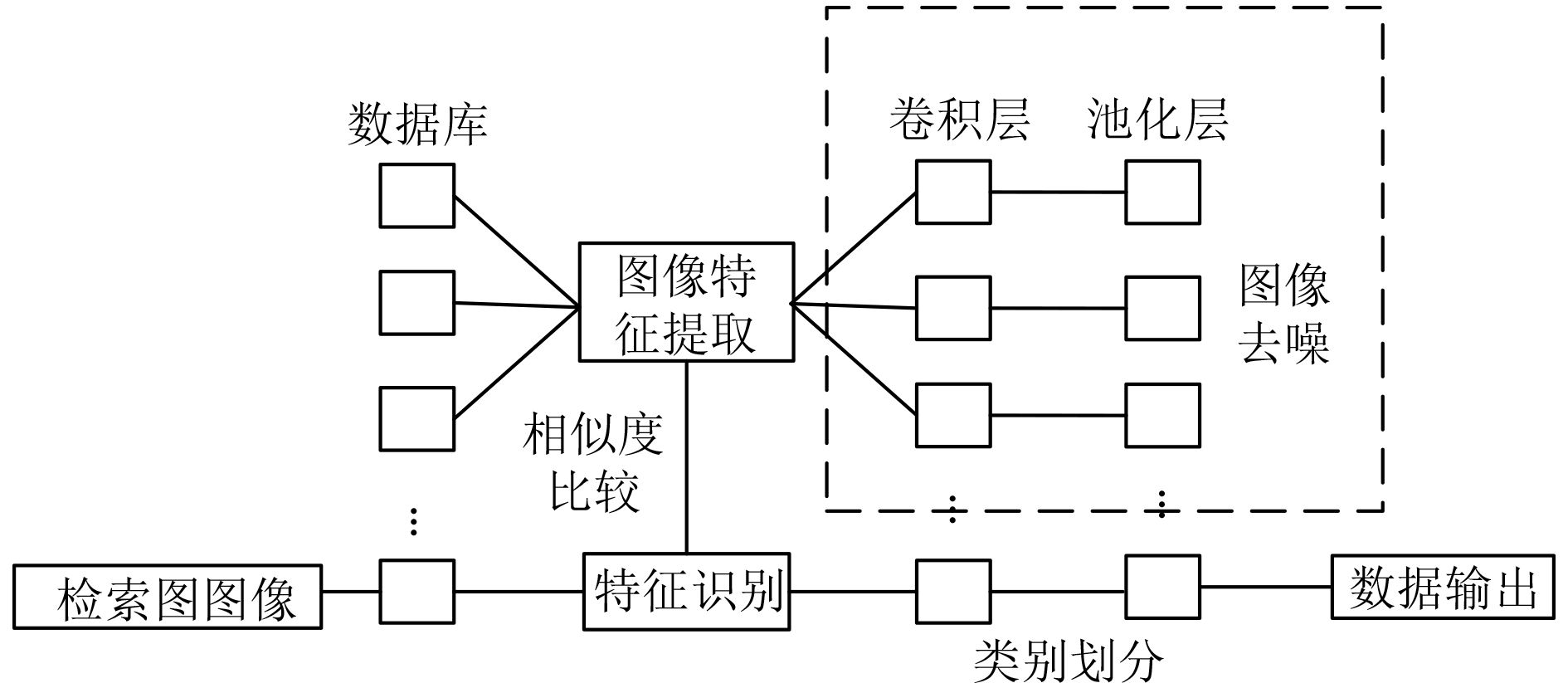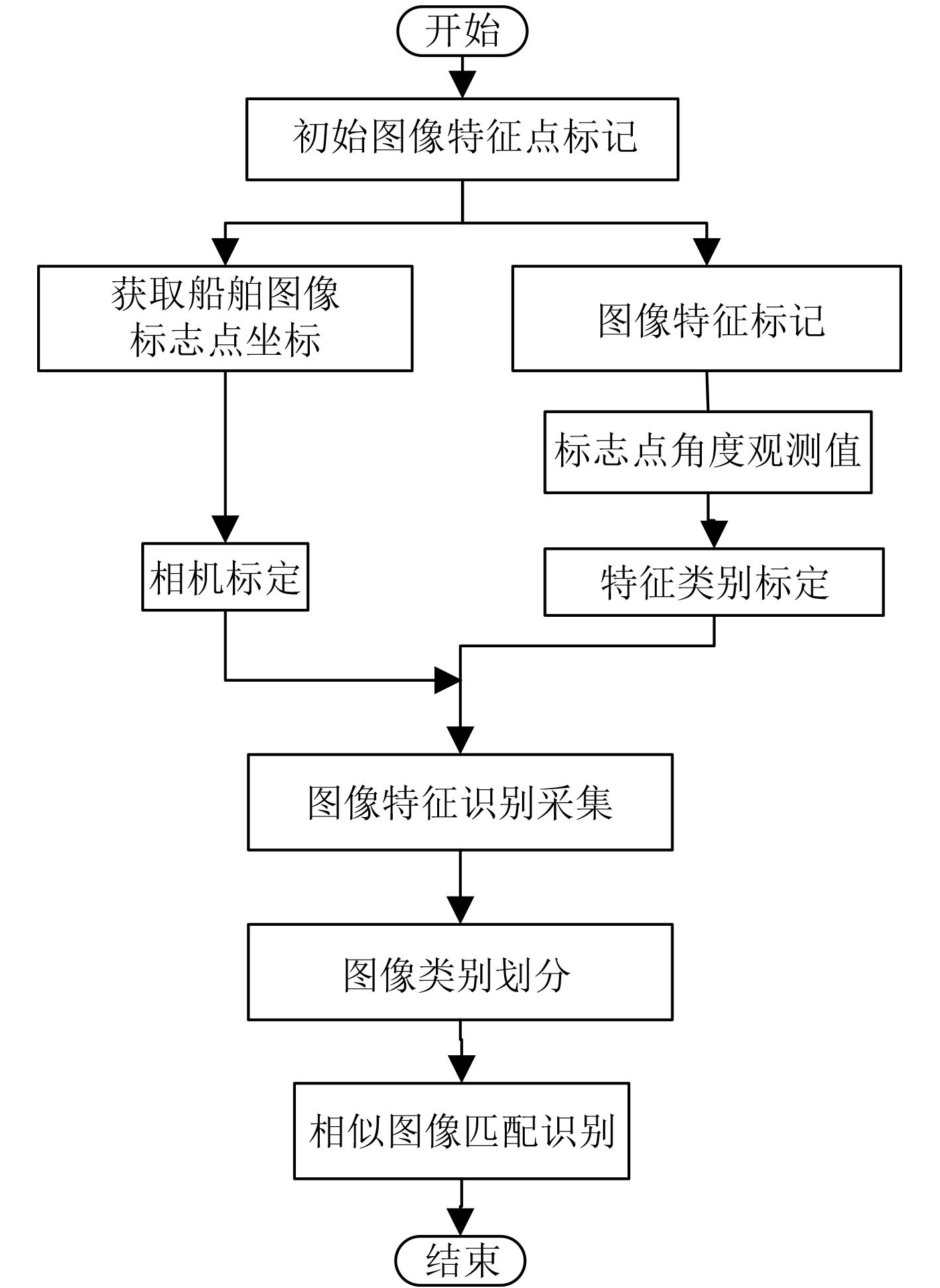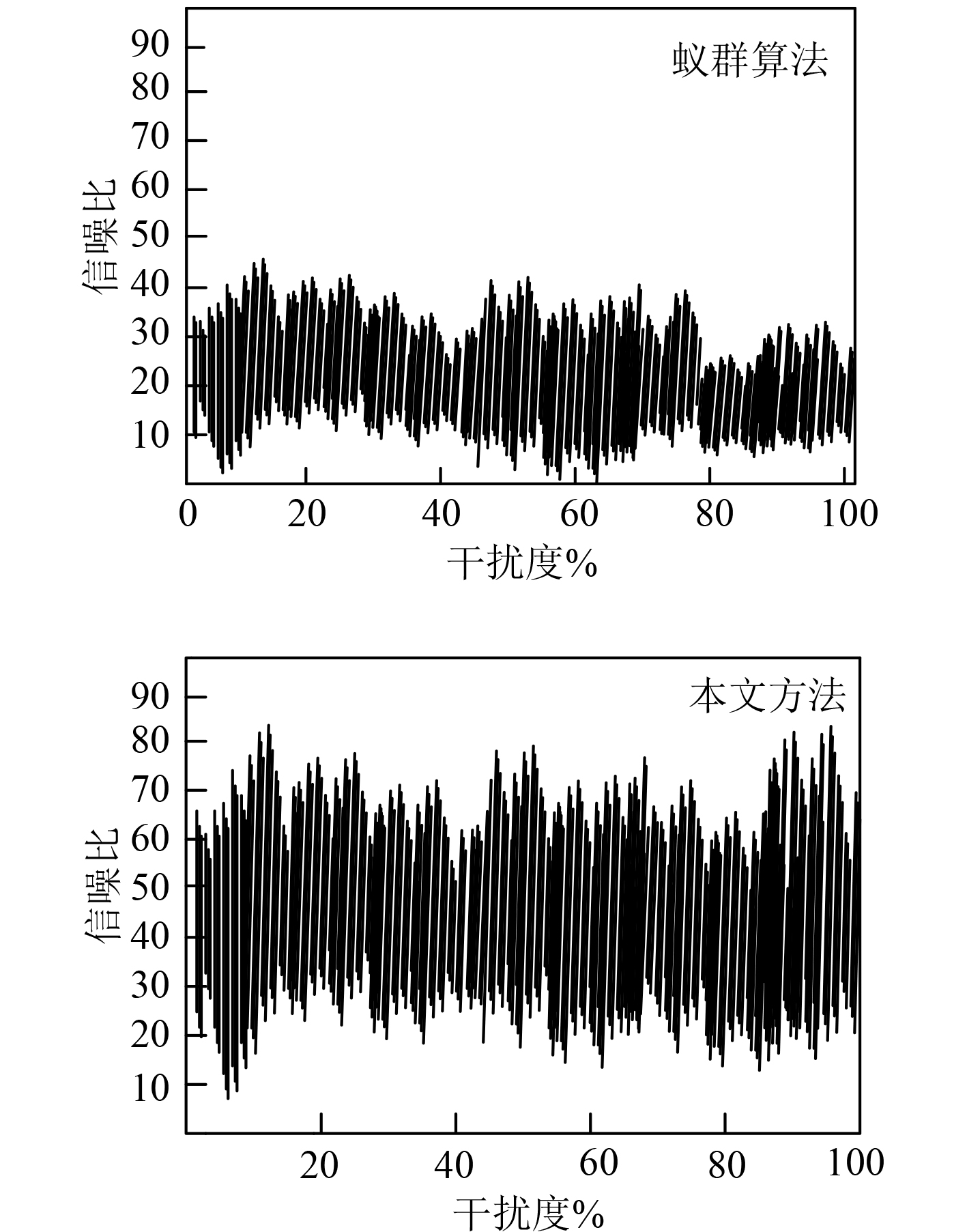﻿ 基于卷积神经网络的船舶图像增强研究
 舰船科学技术2022, Vol. 44Issue (7): 186-189    DOI: 10.3404/j.issn.1672-7649.2022.07.039PDF

Research on ship image enhancement based on convolutional neural network
CHEN Yan
School of Information and Communication Engineering, North University of China, Taiyuan 030051, China
Abstract: In order to better identify the ship image information, a ship image enhancement method based on convolution neural network is proposed. The convolution neural network technology is used to model the ship image features, collect the ship operation image, reduce the noise of the collected data, and then repair the image defects and reverse restore the image based on the image color features, So as to obtain high-quality ship images. Finally, experiments show that the proposed ship image enhancement method based on convolutional neural network can effectively improve the display quality of ship image and meet the design goal of propagation image enhancement.
Key words: convolutional neural network     ship image     image enhancement
0 引　言

1 基于卷积神经网络的船舶图像增强方法 1.1 船舶图像特征采集图 1 卷积神经网络结构 Fig. 1 Convolutional neural network structure图 2 船舶图像特征提取方法 Fig. 2 Ship image feature extraction method

 ${I_2}(x,y) = \prod {p - \left( {{I_1}{{ - }}f(x,y)} \right)} 。$ (1)图 3 图像采集及特征匹配流程 Fig. 3 Image acquisition and feature matching process
1.2 船舶图像去噪算法

 ${C_\psi } = {I_2}(x,y){{ - }}\int_R {\frac{{|\hat \psi (\omega ){|^2}}}{{{\rm{d}}f(x,y)}}} - p 。$ (2)

 ${\psi _{a,b}}(t) = \frac{1}{{{I_1}\sqrt {|p{\text{ - }}a|} }}\hat \psi (\omega )，$ (3)

 $s(t) = x(t) + {C_\psi }{{ - }}{\psi _{a,b}}(t)，$ (4)

 $\overline n = \frac{1}{p}s(t)\sum\limits_{\left( {j,k} \right) \in T} {{g^q}\left( {j,k} \right)} - t，$ (5)

 $F\left( {y,z,\alpha ,\beta } \right) = \left\{ \begin{gathered} {i_1}，\begin{array}{*{20}{c}} {}&{y\sin \alpha + z\cos \alpha > \overline n } ，\end{array} \hfill \\ {i_2}，\begin{array}{*{20}{c}} {}&{y\sin \alpha + z\cos \alpha \leqslant \overline n } 。\end{array} \hfill \\ \end{gathered} \right.$ (6)

 $\gamma = s(t)\sum\limits_{j = 1}^{45} {\overline n {\text{ + }}{\psi _{a,b}}(t)/} F\left( {y,z,\alpha ,\beta } \right) 。$ (7)

1.3 船舶图像增强的实现

 ${h_{W,b}}(x) = {W_T} - \gamma F\left( {y,z,\alpha ,\beta } \right) 。$ (8)

2 实验结果分析图 4 干扰环境下图像信噪比对比检测结果 Fig. 4 Comparison detection results of image signal-to-noise ratio under interference environment